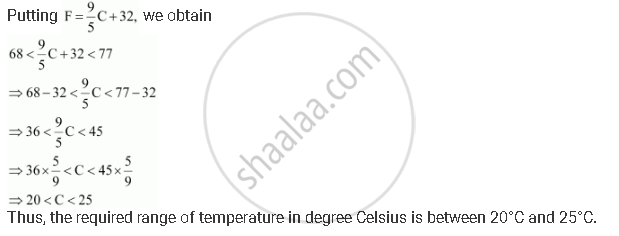CBSE (Arts) Class 11CBSE
Share

# A Solution is to Be Kept Between 68°F and 77°F. What is the Range in Temperature in Degree Celsius (C) If the Celsius/Fahrenheit (F) Conversion Formula is Given by F= 9/8 - CBSE (Arts) Class 11 - Mathematics

ConceptGraphical Solution of Linear Inequalities in Two Variables

#### Question

A solution is to be kept between 68°F and 77°F. What is the range in temperature in degree Celsius (C) if the Celsius/Fahrenheit (F) conversion formula is given by F= 9/8 C + 32 ?

#### Solution

Since the solution is to be kept between 68°F and 77°F, 68 < F < 77Is there an error in this question or solution?

#### APPEARS IN

NCERT Solution for Mathematics Textbook for Class 11 (2018 to Current)
Chapter 6: Linear Inequalities
Q: 11 | Page no. 132

#### Video TutorialsVIEW ALL 

Solution A Solution is to Be Kept Between 68°F and 77°F. What is the Range in Temperature in Degree Celsius (C) If the Celsius/Fahrenheit (F) Conversion Formula is Given by F= 9/8 Concept: Graphical Solution of Linear Inequalities in Two Variables.
S